# Reasoning with Equations and Inequalities

##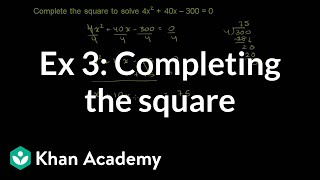By Khan Academy

Sal solves the equation 4x^2+40x-300=0 by completing the square.265# Understanding inequalities and equations

##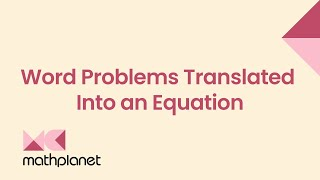By MathPlanetVideos

Margaret is paying \$1,500 in rent for her apartment every month. Margaret won \$12,000 in Vegas and wondering how many rent she can afford to pay with this winning?# Composing equations and inequalities

##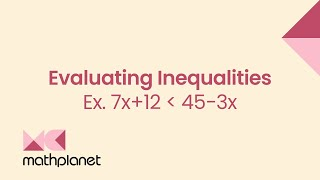By MathPlanetVideos

Is 3 a solution to 7x+12<45−3x# Modeling with one-variable equations and inequalities

##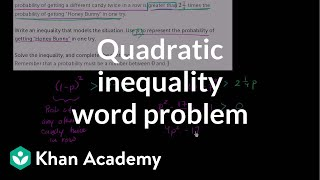By Khan Academy

Sal models a context that concerns a candy vending machine. The model turns out to be a quadratic inequality.# Modeling with one-variable equations and inequalities

##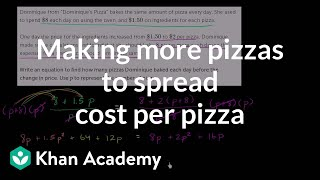By Khan Academy

Sal models a context that concerns the number and price of pizza slices. The model turns out to be a rational equation.# Modeling with one-variable equations and inequalities

##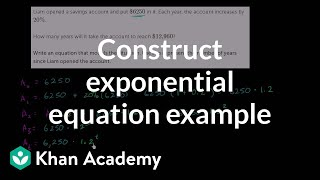By Khan Academy

Sal models a context that concerns a bank savings account. The model turns out to be an exponential equation.# Absolute value inequalities | Linear equations | Algebra I | Khan Academy

##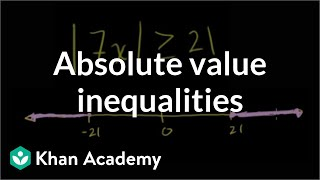By Khan Academy

This video lecture series on Worked Examples in Algebra from Khan Academy includes Solving Equations, Solving Word Problems, Solving for a variable, Absolute Value and Number Lines, Patterns in Sequences, Functional Relationships, Domain and Range, Rate Problems, Linear Functions, Slope of a Line, X and Y intercepts, Equation of a Line, Parallel Lines, Perpendicular Lines, Solving Inequalities and more...# Graphing solutions to two-variable linear equations

##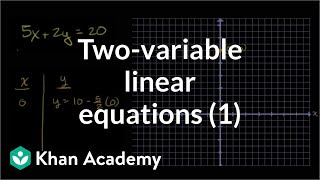By Khan Academy

Plotting (x,y) relationships# Extraneous solutions to radical equations

##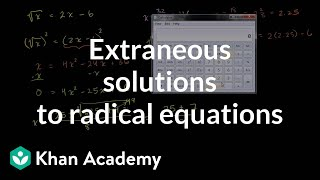By Khan Academy

Sal explains what square-root equations are, and shows an example of solving such an equation and checking for extraneous solutions.# DEPRECATED Extraneous solutions to rational equations

##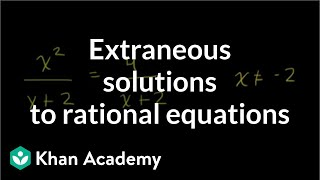By Khan Academy

Sal solves the following equation and eliminates the resulting extraneous solution: (x^2)/(x+2)=4/(x+2).# One-step multiplication and division equations with fractions and decimals

##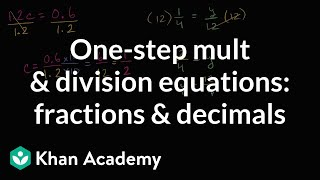By Khan Academy

Learn how to solve equations in one step by multiplying or dividing a number from both sides.ï¿½ï¿½ï¿½ï¿½ï¿½ï¿½ï¿½ï¿½ï¿½ï¿½ï¿½ï¿½These problems involve decimals and fractions.# Grade 7 Math - Modeling Using Equations or Inequalities

##By Lumos Learning

Using the Lumos Study Programs, parents and educators can reinforce the classroom learning experience for children and help them succeed at school and on the standardized tests. Lumos books, dvd, eLearning and tutoring are used by leading schools, libraries and thousands of parents to supplement classroom learning and improve student achievement in the standardized tests.# Grade 6 Math - Solving One-Step Equations 3

##By Lumos Learning

Using the Lumos Study Programs, parents and educators can reinforce the classroom learning experience for children and help them succeed at school and on the standardized tests. Lumos books, dvd, eLearning and tutoring are used by leading schools, libraries and thousands of parents to supplement classroom learning and improve student achievement in the standardized tests.# Grade 6 Math - Solving One-Step Equations 2

##By Lumos Learning

Using the Lumos Study Programs, parents and educators can reinforce the classroom learning experience for children and help them succeed at school and on the standardized tests. Lumos books, dvd, eLearning and tutoring are used by leading schools, libraries and thousands of parents to supplement classroom learning and improve student achievement in the standardized tests.# Find the mistake in solving one-step equations

##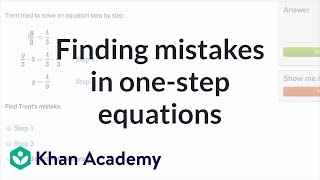By Khan Academy

Learn how to spot mistakes in someone else's work as they attempt to solve one-step equations.# Writing one-step equations word problems

##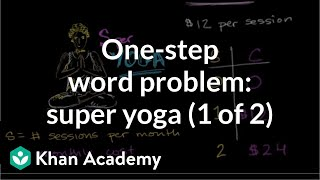By Khan Academy

Using information from the Super Yoga word problem, explore all the possible combinations and create equations which express the possibilities. Let's figure out which plan is best!# Writing one-step equations word problems

##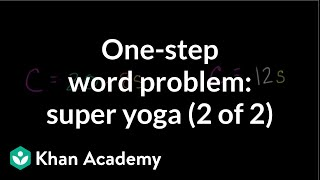By Khan Academy

If you're coming to this video before seeing the previous one, back up! Otherwise, we're solving one-step equations to learn which Super Plan is the best value for our budget.# Grade 6 Math - Solving One-Step Equations 1

##By Lumos Learning

Using the Lumos Study Programs, parents and educators can reinforce the classroom learning experience for children and help them succeed at school and on the standardized tests. Lumos books, dvd, eLearning and tutoring are used by leading schools, libraries and thousands of parents to supplement classroom learning and improve student achievement in the standardized tests.# Writing one-step equations word problems

##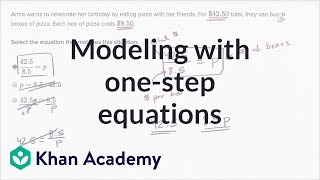By Khan Academy

Learn how to write basic equations to model real-world situations.# Algebra - Equations on a Balance Scale (6-EE-A-2, 6-EE-B-5, 6-EE-B-6)

##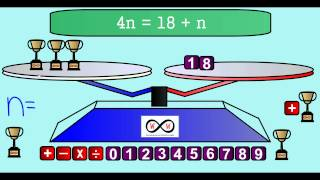By Worksheets and Walkthroughs

This video walkthrough lesson guides you through the process of using a balance scale and manipulatives as visual representations of an equation with a variable representing an unknown value. The solution is also represented as a tape diagram/model drawing. A story problem is also given to provide a meaningful context. (6-EE-A-2, 6-EE-B-5, 6-EE-B-6)